# Supervised learning problems, statistical thinking, and other banal concerns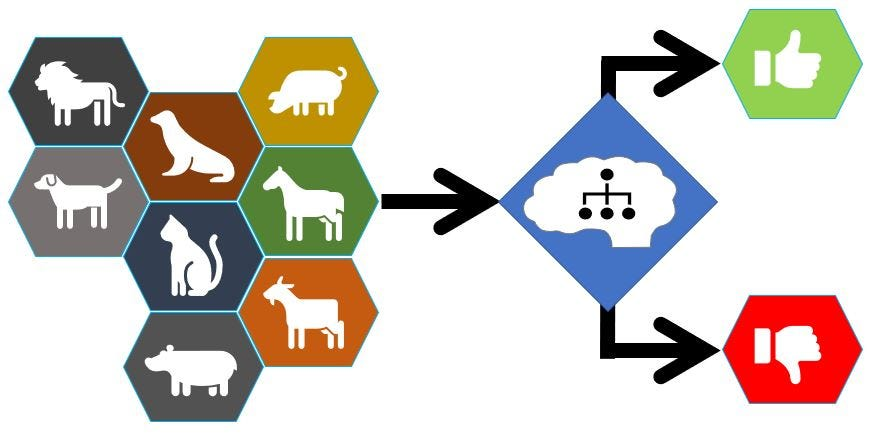Image by author

he supervised learning problems generally fall into three categories: binary classification, multiclass classification, and the last, regression problems.
With binary classification, there are only two possible outcomes, generally, yes or no. With multiclass classification, the result can have an infinity of possible categories, always more than two. Unlike binary and multiclass classification, regression problems tend to have a continuous solution. This last group of problems looks for trends instead of trying to classify the outcome into different groups.
Let’s explain better the differences between these three categories with some examples. The binary classification is used to solve problems where the answer can be one of two values, for instance, whether a photo has a dog: yes or not. But if the idea is to answer the dog’s breed, then it is a multiclass classification problem where each breed is a class. However, if the answer sought is the dog’s age, which is a continuous value between 0 and usually near 12, then it is a regression problem.
In the three cases presented above, a large number of images train the model. In the first case, the training photos have a “is a dog” or “is not a dog” label. In the second case, they have Beagle, Dalmatian, Poodle, Bulldog, Chihuahua, or any other breed as a label. In the last case, the dog’s age will be the label used in the images.

After knowing the type of problems that can be solved by machine learning, it remains to be answered how. There are several types of algorithms, some more suitable than others to answer specific questions. Selecting the most appropriate algorithm to solve a problem is not an easy task. It depends on the type of situation, the data we have access to, and the amount of them, the training power, but above all, the model’s generalization capacity trained in a limited number of data to predict the outcome of a new event.

“All generalizations are false, including this one.”
Mark Twain

Very similar types of machine learning algorithms can solve the three previously mentioned supervised categories of learning problems. Let’s check some of these algorithms using some examples again.

# Decision tree

The decision tree algorithm is one of the most used Machine Learning algorithms for classification problems. It is a user-friendly algorithm. With it, it is possible to show how the machine makes predictions by creating a graphical tree, thus becoming easy to understand.
Starting with the tree’s root node, a specific feature is tested in each tree’s node. Depending on the outcome, it follows different branches on the tree, trying new nodes where other features are tested until reaching a terminal node. The outcome of this terminal node is the prediction result of the model.
Let’s understand how this works using an imaginary loan approval example. It is possible to create a dataset using historical data. As the target of our prediction is used the loan status. Credit History, Income, and Loan Amount will be our Features.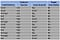Decision tree sample data

On the first node of the tree, it checks the customer credit history. In our example, we have four customers with good credit history, two of them with a target equal to “No” and another two with a target equal to “Yes.”
Then, it checks the customer’s income. There are two customers with good credit history and high income in our sample data, and these two customers have the target labeled as “Yes.”
Finally, it used the requested loan amount. Based on our data, the tree should consider high provability to approve loans to customers with good credit history, high income, and that request a big amount of credit.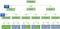Loan approval decision tree. Image by author

The feature importance and the subsequent order to test the tree features on each node is decided based on the Gini Impurity or Gain Information criteria. These two criteria identify the degree of uncertainty for each feature. When building the tree, the model must first test the resources with the greatest gain of information (i.e., least uncertainty). This dependence between the data uncertainty and the tree structure is a disadvantage of this algorithm since a small change in the data can affect the tree structure.

# Random Forest

The Random Forest Algorithm uses the output of multiple Decision Trees, randomly created, to generate the model’s final result. Each tree is made with a random subset of features and data, and subsequently with a different structure. In the end, this algorithm combines the output of each decision tree to generate the final result.

The process used to combine the multiple individual tree outputs to get a final result is called Ensemble Learning. Ensemble Learning assumes that the results obtained from consulting a diverse group of models are likely to be better than the results obtained from a single model. The point here is how the result of the several models can generate a single final output. There are several techniques for it. The most simples ones are considered the most frequent result (i.e., most voted) or the average between the several results.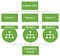Random Forest. Image by author

# k-Nearest Neighbor

A common supervised machine learning algorithm for multiclass classification is k-Nearest Neighbor. This algorithm assumes that similar things are near to each other. The idea is to compare the distances between the new element that we want to predict and the known elements. Each element represents a point in a multidimensional space, where each element’s feature is a spatial dimension. Euclidean distance or Manhattan distance determined how near is the new element from its neighbors. In the end, elements of the same class should have the shortest distance between them. Considering the class to which belong to the k elements nearest to the new element, we can deduce which class the new element belongs. Minimizing the distance is a crucial part of this algorithm. The closer you are to your nearest neighbors, the more likely you are to be accurate.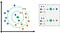k-Nearest Neighbor. Image by author

The algorithm has the disadvantage of requiring an enormous calculation power since, for each new sample, it is necessary to iterate with the training data again. However, recommendation systems widely use this algorithm.

# Naive Bayes

Another family of supervised learning models is the Naive Bayes family of classifiers. This algorithm is based on Bayes’ theorem, which is mostly used for binary or multiclass classification. It’s called naive because it has as a basis the assumption that all features are independent of another. In practice, this is not often the case because features are usually somewhat correlated. Since the statistics of each feature are calculated independently, learning a Naive Bayes classifier can be very fast. However, the penalty for this efficiency is that the prediction performance of Naive Bayes can be a bit worse than other more sophisticated algorithms.

This algorithm uses the independent probability that each feature belongs to a class without considering other features to predict the model’s result. Let’s illustrate this using our imaginary loan approval example, considering only the “Income” feature: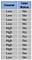Income data sample

First, it is necessary to get the frequency table: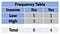Frequency table

Using the frequency table, get the “Likelihood of Evidence” of the “Income” feature: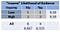Likelihood table

With this training data, it can be concluded that, for eight denied loans, five have “Low” income. In other words, the likelihood probability of customers with “Low” income has a loan denied is 5/8 = 0.625. The notation of this is:

• P(“Income” = “Low” | “Loan Status” = “No”).

Likewise, from twelve requested loans, eight were denied, and six have low income, resulting in:

• P(“Income” | “Loan Status” = “No”) = 8/12 = 0.667.
• P(“Income” = “Low”) = 6/12 = 0.50.

Applying Bayes’ theorem, we can calculate the probability of having the loan denied when the customer has a low income: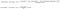Bayes’ theorem application

This results in a probability of 0.8375 of having the loan denied.
Similarly, the probability of the remaining feature components can be calculated for the different outcome classes. Finally, the class that gets the highest probability will be the predicted class.

# Regression

Machine learning uses several types of regression algorithms. Among them are: Linear Regression, Logistic Regression, and Polynomial Regression.

Linear Regression uses a linear function to describe the relationship between the outcome and its inputs. An input value X associated with an output value Y can be represented using a point in a Cartesian coordinate system. In this way, a set of points in the plane represents a significant number of occurrences. Linear Regression consists in finding the straight line that best fits with this set of points. The best-fit line is called the regression line.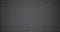Linear regression. Image Image by author

Predicting the value Y using a value of X is just a matter of applying the linear equation of the identified regression line:

Where m is the slope of the identified line, and b is the value of Y when X is equal to zero (i.e., the point where the line cuts the Y-axis).
But what is meant by “best-fitting line”? Firstly, it is necessary to measure the difference between the observed point and the predicted value (i.e., the point in the line).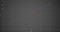Difference between the predicted value and the real one. Image by author

Secondly, the square of the difference between the predicted value and the real one is calculated for each point. Finally, the average of this value is inferred, resulting in an indicator called Mean Squared Error. The most used criterion to find the best-fitting line is the line that has the minimum Mean Squared Error.

However, it is not always possible to find a straight line that follows most points. Sometimes the line that best fits is a curve that results from an nth degree polynomial. In these cases, the Polynomial Regression algorithm is applied, transforming the equation of the line to:

Now imagine that instead of having a single feature X, there are two features: X and Z. In this case, the line becomes a surface, and the goal becomes to identify the surface that best fits all points in a three-dimensional space.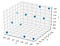Three-dimensional space. Image by author

Logistic Regression differs from the previous by having a categorical variable as its output, instead of a continuous value. The output of this algorithm is the probability of the sample belonging to one class versus another.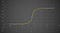Logistic Regression. Image by author

Similar to Linear Regression, a line’s equation describes the relationship between features and output. However, in this case, the result to get is the probability of the equation result belonging to a specific class. The way to evaluate this probability is by using a logistic equation that returns a value between 0 and 1. A Logistic equation is an S-shaped curve called a “sigmoid curve.” This curve is represented using the following equation:

Where y is the output of the linear function, and y0 is the value of the sigmoid’s midpoint.

There are many other algorithms. This post intends to describe the most relevant and easy to understand algorithms in a very superficial way.

“Statistical thinking will one day be as necessary a qualification for efficient citizenship as the ability to read and write.”

H.G. Wells 

I hope that this contribution helps start the long path of improvement for all who want to develop their “Statistical thinking.”

# References

 Mankind in the Making | H.G. Wells | The Project Gutenberg (originally published in 1903)

Passionate about Machine Learning, Python, React, JavaScript, Java, Laravel, and many other things.

## More from Carlos Rodrigues

Passionate about Machine Learning, Python, React, JavaScript, Java, Laravel, and many other things.